## Power Pivot, Part 4 of 5: DAX, Formulas, and Functions

#### Watch trailer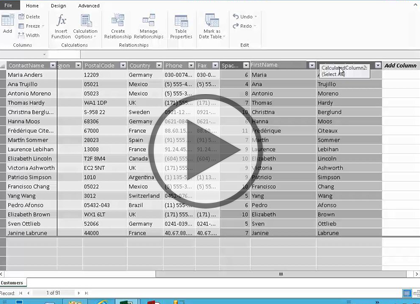#### Course at a glance

Included in these subscriptions:

• Dev & IT Pro Video
• Dev & IT Pro Power Pack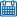Release date 8/18/2014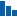Level Intermediate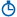Runtime 2h 6m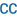Closed captioning Included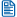Transcript Included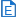eBooks / courseware Included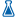Hands-on labs N/A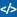Sample code Included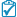Exams Included#### Course description

In this course we’ll start by covering what is DAX, why DAX is so important and how it integrates with Power Pivot 2013. Why use DAX, there is a reason why it has been mentioned over and over for quite some time with Power Pivot and SQL. We’ll discuss the rhymes and reasons of it as well as the history of DAX followed by DAX functions and references. Then we’ll cover the DAX functions category. These categories are Text, Filter, Information, Logical, Date and Time, Math and Trig, and more.

#### Prerequisites

This course assumes that the users have an understanding of Microsoft Excel and how databases function. You should have viewed the Power Pivot: Data Models, Power Pivot: Basic Concepts and Power Pivot: Dashboards and Power View courses before viewing this course.

#### Learning Paths

This course is part of the following LearnNowOnline SuccessPaths™:

#### Meet the expertDoug Ortiz is an independent consultant whose skillset encompasses multiple platforms including .NET, SharePoint, Office, SQL Server, and Business Intelligence. Doug has a master&rsquo;s degree in relational databases and over 20 years of experience in IT, over half of which have been within .NET and SharePoint. His roles both in and outside of SharePoint have ranged from architecture, implementation, administration, disaster recovery, migrations, development, and automation of information systems.

#### DAX and Formulas

##### Key Concepts (13:53)
• Introduction (00:25)
• What is DAX? (00:28)
• DAX Data Types (00:42)
• Context (00:31)
• Row Context (00:21)
• Filter Context (00:33)
• DAX Formula Syntax (02:22)
• Why DAX? (01:29)
• History (00:20)
• Where are DAX Formulas used? (00:27)
• Reference by Category (00:37)
• FILTER Functions (00:44)
• Information Functions (00:37)
• Logical Functions (00:07)
• Statistical Functions (00:22)
• Data and Time Functions (00:17)
• Time Intelligence Functions (00:23)
• Math and Trig Functions (00:16)
• Text Functions (00:17)
• Other Functions (00:15)
• DAX Function Reference (01:49)
• Summary (00:21)
##### Creating Formulas (18:33)
• Introduction (00:16)
• Calculated Fields (01:28)
• Demo: Calculated Fields (05:16)
• Calculated Columns (01:19)
• Demo: CC - FIND (04:55)
• Demo: CC - FIND, LEN (03:55)
• Calculated Field or Column? (01:12)
• Summary (00:09)
##### TextFunctions (10:04)
• Introduction (00:11)
• Text Functions (00:50)
• Demo: TF - CONCATENATE, LEN (04:01)
• Demo: TF - UPPER, SUBSTITUTE (04:15)
• Summary (00:44)
##### Filter Functions (20:11)
• Introduction (00:15)
• Filter Functions (00:28)
• Demo: FF - COUNTROWS (05:07)
• Demo: FF - DISTINCT, RELATED (04:02)
• Demo: FF - ISFILTERED (05:08)
• Demo: FF - CALCULATE (04:28)
• Summary (00:41)

#### Functions

##### InformationFunctions (10:39)
• Introduction (00:13)
• Information Functions (00:47)
• Demo: IF - PATH, PATHLENGTH (05:10)
• Demo: IF – LOOKUPVALUE,ISBLANK (04:00)
• Summary (00:28)
##### Logical Functions (10:08)
• Introduction (00:21)
• Logical Functions (00:30)
• Demo: LF - SWITCH, IF (04:59)
• Demo: LF - AND, OR, IFERROR (03:50)
• Summary (00:25)
##### StatisticalFunctions (17:01)
• Introduction (00:26)
• Statistical Functions (00:29)
• Demo: SF – COUNTs (04:53)
• Demo: SF – COUNTs, MAX, MIN (04:56)
• Demo: SF – TOPN, GENERATE (05:33)
• Summary (00:42)
##### DateAndTimeFunctions (13:01)
• Introduction (00:27)
• Date and Time Functions (00:35)
• Demo: DaTF – EDATE, EOMONTH (02:43)
• Demo: DaTF – Quarterly (04:13)
• Demo: DaTF – PivotTable (04:27)
• Summary (00:34)
##### MathAndTrigFunctions (12:43)
• Introduction (00:19)
• Math and Trig Functions (00:43)
• Demo: MaTF – Math I (03:51)
• Demo: MaTF – Math II (02:06)
• Demo: MaTF – Calc Fields (04:56)
• Summary (00:45)